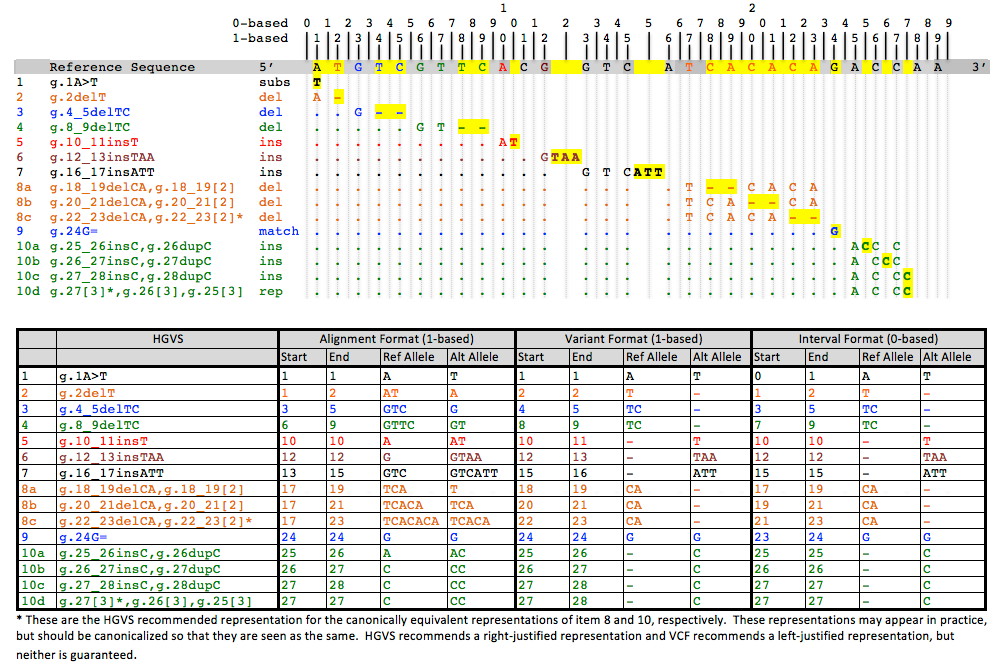# Coordinate Numbering

The explanation and background behind the decisions made to represent allele reference coordinates using a zero-based interval approach.

The Data Model Working Group considered three methods for the numbering of coordinates:

1. The Alignment Method: Based on the numbering used in VCF.
2. The Variant Method: Based on the numbering used in HGVS expressions.
3. The Interval Method: Based on numbering intervals as in BED files.

In the Alignment Method, the reference allele is defined as the subsequence that begins at the base numbered "start" and goes to the base numbered "end", both inclusive. A single base will have equal start and end. As in a VCF, insert or delete alleles will usually share the first base of the reference alleles, which is not actually part of the variable site, but is used to help locate the variable site.

In the Variant Method, the reference allele is not computable from the start and end positions, but is an integral part of the definition of the coordinate. If the location is a range of bases, then start, end and ref are defined as in the alignment model. However, if the interval between two bases is the location, then start is the position of the first base, end = start + 1, and ref is an empty string. This method has the advantage that only variable bases are included in the definition of the alleles, but it has the disadvantage that two coordinates may have the same start and end, but in one case refer to a pair of bases, and in the other case refer to the interval between the two bases.

In the Interval Method, often called zero-based counting, positions denote the intervals between bases, rather than referring to the bases themselves, with a position of 0 denoting the interval before the first base, 1 the interval between the first and second base, and so on. With this counting, the reference allele is again computable as the bases that lie between the start and end intervals. In the case of a single base reference allele, the end position will be start + 1. If start = end = i, then the position is the interval after the i-th base. Any ContextualAllele that has such a coordinate would therefore be an insertion. The interval method has the advantages that only variant bases are included in the allele definitions, that the reference allele is completely computable, as well as consistency in the meaning of start and end. Furthermore, the interval method is capable of representing the alleles of a snp and a deletion of the varying base using the same allele coordinate, which the alignment method cannot.

The table below shows the differences in representation for reference coordinates in the methods described above. Although Alt is not part of the coordinate, it is included here to illustrate the manner in which alleles would make use of the reference coordinates in different circumstances.## Intronic Coordinates

Intronic regions are often designated with respect to a transcript; however introns are not actually part of the transcript sequence. This means that extra coordinate information must be added to the basic method outlined above. Further, it is often convenient to allow intronic coordinates to be counted from either end of the intron, so that the distance to the nearest exon/intron boundary is clear. We specify intronic coordinates using three additional values: an intronic start, an intronic end, and a direction, which allows the start and end to be counted from either side of the intron. The following tables illustrate this method:

Sequence   A   C   T   g   c   a   c   t   a   C   A
Transcript Numbering 0   1   2   3                       3   4   5
Intronic Left (relative to the T)             0   1   2   3   4   5   6
Intronic Right (relative to the C)             6   5   4   3   2   1   0

In this example, uppercase bases represent exonic sequence, and lowercase sequences represent intronic bases. In the table, lowercase and uppercase are used to aid in understanding, but the actual transcript reference sequence would not include the intronic bases. The transcript numbering does not include the intronic bases; note that the entire intron is in the transcript interval numbered 3.

offsetstart, end and direction are understood to be attributes of a related Position. If both values are empty in the table, then no such related entity exists.

Example Type start end ref offsetStart offsetEnd offsetDirection
Exonic 1 2 C
Intronic Left 3 3 c 1 2 +
Intronic Right 3 3 t 1 2 -
Intronic Long 3 3 cac 1 4
Spanning 1 3 CTgc   2 +